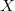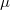Want to share your content on R-bloggers? click here if you have a blog, or here if you don't.

I have a rather simple question regarding the use of MSE as opposed to MAD and MAPE. If the parameters of a time series model are estimated by minimizing MSE, why do we evaluate the model using some other metric, e.g., MAD and MAPE. I could see that MAPE is not scale dependent. But MAPE is a percentage version of MAD. So why don’t we use the percentage version of MSE?

MSE (mean squared error) is not scale-free. If your data are in dollars, then the MSE is in squared dollars. Often you will want to compare forecast accuracy across a number of time series having different units. In this case, MSE makes no sense. MAE (mean absolute error) is also scale-dependent and so cannot be used for comparisons across series of different units. The MAD (mean absolute deviation) is just another name for the MAE.

The MAPE (mean absolute percentage error) is not scale-dependent and is often useful for forecast evaluation. However, it has a number of limitations. For example,

1. If the data contain zeros, the MAPE can be infinite as it will involve division by zero. If the data contain very small numbers, the MAPE can be huge.
2. The MAPE assumes that percentages make sense; that is, that the zero on the scale of the data is meaningful. When forecasting widgets, this is ok. But when forecasting temperatures in degrees Celsius or Fahrenheit it makes no sense. The zero on these temperature scales is relatively arbitrary, and so percentages are meaningless.

It is possible to have a percentage version of MSE, the Mean Squared Percentage Error, but this isn’t used very often.

The MASE (mean absolute scaled error) was intended to avoid these problems.

For further discussion on these and related points, see Hyndman & Koehler (IJF, 2006). A preprint version is also available.

Also, suppose we have a lognormal model, where the estimation is done on the log-transformed scale and the prediction is done on the original, untransformed scale. One could either predict with the conditional mean or the conditional median. It seems to me that you would predict with the mean if the MSE is your metric, but you would predict with the median if the MAD is your metric. My thought is that the mean would minimize MSE, while the median would minimize MAD. So whether you use the mean or the median depends on which metric you use for evaluating the model.

In most cases, the mean and median will coincide on the transformed scale because the transformation should have produced a symmetric error distribution. I would usually estimate with the MSE because it is more efficient (assuming the errors look normal). It might help to estimate with the MAD if there are outliers, but I would prefer to explicitly deal with them.

When forecasting on the original, untransformed scale, the simple thing to do is to back-transform the forecasts (and the prediction interval limits). The point forecasts will then be the conditional median (assuming symmetry on the transformed scale), and the prediction interval will still have the desired coverage.

To get the conditional mean on the original scale, it is necessary to adjust the point forecasts. Ifis the variable on the log-scale andis the variable on the original scale, thenwhereis the point forecast on the log-scale andis the forecast variance on the log-scale. The prediction interval remains unchanged whether you use a conditional mean or conditional median for the point forecast.

Occasionally, there may be some reason to prefer a conditional mean point forecast; for example, if you are forecasting a number of related products and you need the point forecasts to sum to give the forecast of total number of products. But in most situations, the conditional median will be suitable.

In R, the `plot.forecast()` function (from the forecast package) will back-transform point forecasts and prediction intervals using an inverse Box-Cox transformation. Just include the argument `lambda`. For example:

 ```fit <- ar(BoxCox(lynx,0.5)) plot(forecast(fit,h=20), lambda=0.5)```